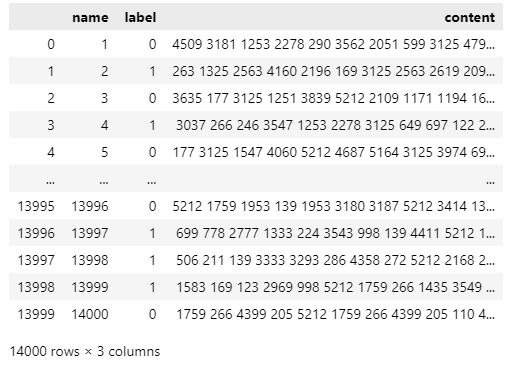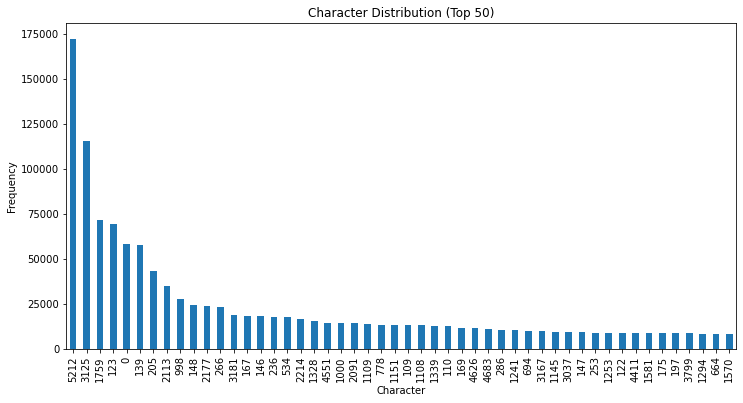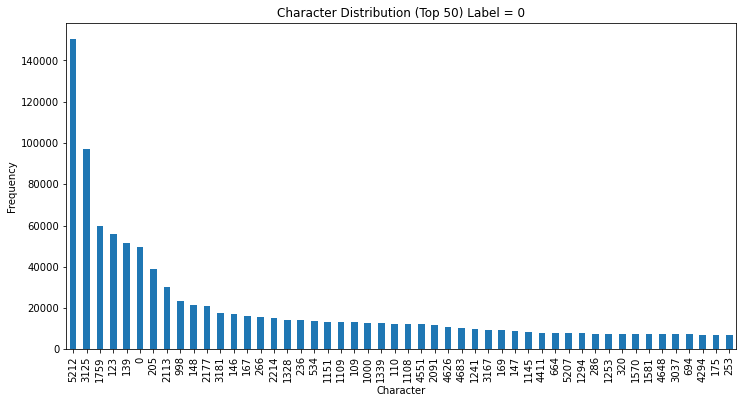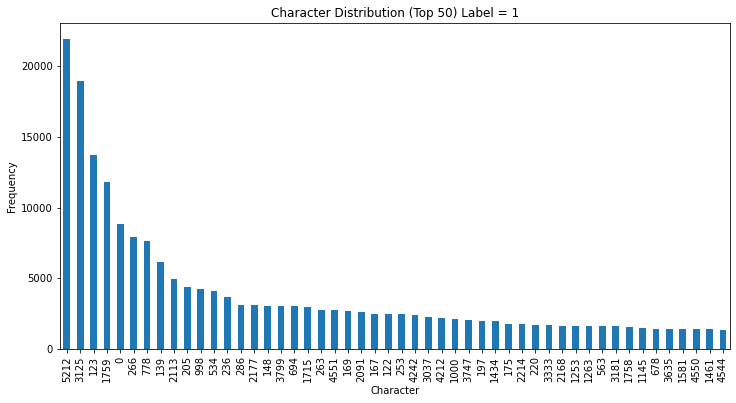##MSIPO技术圈 首页 IT技术 查看内容

2023-07-13

# Coggle 30 Days of ML (23年7月）任务二：数据可视化

#### 任务二：对数据集字符进行可视化，统计标签和字符分布

• 说明：在这个任务中，需要使用Pandas库对数据集的字符进行可视化，并统计数据集中的标签和字符的分布情况，以便更好地理解数据集。
• 实践步骤：
1. 使用Pandas库读取和加载数据集。
2. 使用Pandas的可视化功能，如柱状图或饼图，对数据集的字符进行可视化展示。
3. 使用Pandas的统计功能，如value_counts()方法，统计数据集中的标签和字符的分布情况。

## 数据读取

``````train_data = pd.read_csv('ChatGPT/train.csv')
``````

## 数据可视化``````train_data['char_count'] = train_data['content'].apply(lambda x:len(x.split(' ')))
print(train_data['char_count'].value_counts())

# 绘制字符数量分布柱状图
train_data['char_count'].plot(kind='hist', bins=30, rwidth=0.8)
plt.xlabel('Character Count')
plt.ylabel('Frequency')
plt.title('Distribution of Character Counts')
plt.show()
``````
``````200    13956
176        3
150        3
1          2
188        2
181        2
198        2
184        2
193        2
167        2
177        2
187        2
166        2
81         1
197        1
180        1
196        1
160        1
134        1
199        1
130        1
102        1
142        1
172        1
173        1
171        1
185        1
195        1
154        1
186        1
161        1
Name: char_count, dtype: int64
````````````train_data[train_data['char_count'] != 200]['label'].value_counts()
``````
``````1    30
0    14
Name: label, dtype: int64
``````

## 标签分布可视化

``````# 统计标签分布
label_counts = train_data['label'].value_counts()
print(label_counts)
# 绘制标签分布条形图
plt.bar(label_counts.index, label_counts.values)
plt.xlabel('Label')
plt.ylabel('Count')
plt.title('Label Distribution')

# 添加标签名称
label_names = ['Label 0', 'Label 1']  # 用实际的标签名称替换这些示例名称
plt.xticks(label_counts.index, label_names)

plt.show()
``````
``````0    11836
1     2164
Name: label, dtype: int64
``````## 字符分布统计

``````# 获取字符数量分布数据
char_distribution = train_data['content'].str.split(' ', expand=True).stack().value_counts()

# 绘制前50个字符数量分布柱状图
char_distribution[:50].plot(kind='bar', figsize=(12, 6))
plt.xlabel('Character')
plt.ylabel('Frequency')
plt.title('Character Distribution (Top 50)')
plt.show()
``````### Label为0

``````# 获取字符数量分布数据
char_distribution = train_data[train_data['label']==0]['content'].str.split(' ', expand=True).stack().value_counts()

# 绘制前50个字符数量分布柱状图
char_distribution[:50].plot(kind='bar', figsize=(12, 6))
plt.xlabel('Character')
plt.ylabel('Frequency')
plt.title('Character Distribution (Top 50) Label = 0')
plt.show()
``````### Label为1

``````# 获取字符数量分布数据
char_distribution = train_data[train_data['label']==1]['content'].str.split(' ', expand=True).stack().value_counts()

# 绘制前50个字符数量分布柱状图
char_distribution[:50].plot(kind='bar', figsize=(12, 6))
plt.xlabel('Character')
plt.ylabel('Frequency')
plt.title('Character Distribution (Top 50) Label = 1')
plt.show()
``````